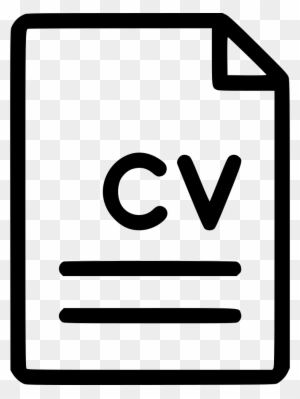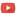KE8QZC | SFW | TSWSyllabus

Homework 1 (solution) ($\S$1.1): pg.10 #7-14 (solve all systems using matrix method only!)
Homework 2 (solution) ($\S$1.2,1.3): pg.21-22 #1,2,7,8; pg.32-33 #5,6,9,10,11,12,13
Homework 3 (solution) ($\S$1.3,1.4): pg. 32-33 #25,26, pg.40-41 #1,2,3,5,7,8,11,12 and the following additional problem:
(A) Solve the matrix equation $A \vec{x}=\vec{b}$ where $A = \left[ \begin{array}{ll} 1 & 0 & 1 \\ 0 & -1 & 1 \\ 0 & 0 & -1 \end{array} \right]$ and $\vec{b}=\left[ \begin{array}{ll} b_1 \\ b_2 \\ b_3 \end{array} \right]$
Homework 4 (solution) ($\S$1.5-1.6) pg.47-48 #1,3,5,7,9,26,27,38,39,40 pg.54 #6
Homework 5 (solution) ($\S$ 1.7-1.8): pg. 60 #1,3,5,7,31,35,37; pg.68 #1,3,5,7,8
Homework 6 (solution) ($\S$1.8,2.1): pg. 68 #13,14,21,23,24; pg.100 #1,2,3,7,9,27 and the following additional problem:
Problem A: Find the image of the square whose corners lie at the points $(0,0),(1,0),(0,1),(1,1)$ in the plane under the linear transformation $$\begin{array}{ll} T \colon \mathbb{R}^{2 \times 1} \rightarrow \mathbb{R}^{2 \times 1} \\ T(\vec{x})=A\vec{x}, \end{array}$$ where $A$ is the matrix $A=\begin{bmatrix} 1 & 5 \\ 0 & 1 \end{bmatrix}.$
Homework 7 (solution) ($\S$2.2): pg. 109 #1,2,5,8,16,17,18,22,24,26,31,38
Homework 8 (solution) ($\S$2.3,2.4,3.1):pg.115 #5,6,7,8,15,16,17,18, pg.121 #1,2,3,4, pg. 167 #1,2,3,4 (compute the determinants any way you wish)
Homework 9 (solution) ($\S$2.5,3.1,3.2,3.3): pg. 129 #1,7,15,16, pg.168 #33,37, pg.175 #29,31,32,40, pg.184 #1,2,19,23
Homework 10 (solution) ($\S$4.1): pg. 195 #1,2,3,6,8,9,11,20,21,22,32,33
Homework 11 (solution) ($\S$4.2): pg.205-207 #1,3,5,15,25,26,29,30,31,32,33,34
Homework 12 (solution) ($\S$4.3): pg. 213 #1,2,3,4,5,21,22,23,28,33,34
Homework 13 (solution) ($\S$4.4): pg. 222 #1,4,7,8,13,14,15,16,18,19
Homework 14 (solution) ($\S$4.5): pg. 229 #1,2,4,6,7,9,12,13,14,22,24,25,27
Homework 15 (solution) ($\S$4.6): pg.237 #1,2,3,4,6,8,9,10,11,12,13,14,16,17,18
Homework 16 (solution) ($\S$5.2,inner products): (note: find all eigenvalues, even complex ones) pg.271 #1,4.
Also do the following additional problems (notes for inner product spaces can be found at a link at the bottom of this page because they do not appear in the book):
Problem A. Find all eigenvalues of the following matrix: $$\begin{bmatrix} 1&0&0&0&0&0&0 \\ 0&5&0&0&0&0&0 \\ 0&0&17&0&0&0&0 \\ 0&0&0&-5&0&0&0 \\ 0&0&0&0&89&0&0 \\ 0&0&0&0&0&\pi&0 \\ 0&0&0&0&0&0&0 \end{bmatrix}.$$ Problem B. Find an eigenvector corresponding to the eigenvalue $\lambda=4$ of the matrix $$\begin{bmatrix} 3&0&-1 \\ 2&3&1 \\ -3&4&5 \end{bmatrix}.$$ Problem C. Find a basis for the eigenspace corresponding to each eigenvalue $\lambda=1,3$ of the matrix $\begin{bmatrix} 3 &0 \\ 2 &1 \end{bmatrix}$.
Problem D. Let $H=(\mathbb{R}^3,\langle \cdot,\cdot \rangle)$ be the inner product space of Example 1. Let $\vec{x}=\begin{bmatrix} 3 \\ 2\\ -1\end{bmatrix}$ and $\vec{y}=\begin{bmatrix} 2 \\ 1 \\ 17\end{bmatrix}$. Compute $\langle \vec{x},\vec{y} \rangle$.
Problem E. Let $H=(\mathbb{P}, \langle \cdot,\cdot \rangle)$ be the inner product space of Example 2. Let $\vec{p}(x)=x-1$, $\vec{q}(x)=x^2$. Compute both of the inner products $\langle \vec{p},\vec{q} \rangle$ and $\langle \vec{p},\vec{p} \rangle$ using integration by parts.
Problem F. Use integration by parts to calculate the antiderivative of $f(x)=\log(x)$. (Hint: use $u=\log x$ and $dv=1$. Also recall that $\dfrac{d}{dx} \log x = \dfrac{1}{x}$)
Problem G. Let $H=(C[0,1],\langle \cdot,\cdot \rangle)$ be the inner product space of Example 3. Let $f(x)=\log(x+1)$ and $g(x)=1$. Calculate $\langle f,g \rangle$. Let $h_1(x)=x^2$ and $h_2(x)=\sin(x)$. Calculate $\langle h_1,h_2 \rangle$ (hint: use integration by parts).
Problem H. Let $H=(\ell^1(\mathbb{R}),\langle\cdot,\cdot\rangle)$ be the inner product space of Example 4. Let $\{a_k\}_{k=0}^{\infty} = \left\{ \dfrac{1}{3^k} \right\}_{k=0}^{\infty}$ and $\{b_k\}_{k=0}^{\infty} = \left\{ \dfrac{1}{7^k} \right\}_{k=0}^{\infty}$. Calculate $\left\langle \left\{ a_k \right\}, \left\{b_k\right\} \right\rangle$ (hint: this is a geometric series). Let $\left\{c_k\right\}=\left\{d_k\right\}=\sqrt{\dfrac{1}{k!}}$. Calculate $\langle c_k,d_k \rangle$ (hint:recall the power series $e^x = \displaystyle\sum_{k=0}^{\infty} \dfrac{x^k}{k!}$).
Problem I. Let $H=(\mathbb{C},\langle\cdot,\cdot\rangle)$ be the inner product space of Example 5. Let $\vec{x}=5+4i$ and $\vec{y}=9-11i$. Compute $\langle \vec{x},\vec{y}\rangle$. Let $z_1=21+16i$ and $z_2=\dfrac{11-5i}{2+i}$. Calculate $\langle z_1,z_2\rangle$ (hint: mutltiply $z_2$ by $1=\dfrac{2-i}{2-i}$ to put $z_2$ into the form $z_2=a+bi$; this is similar to "rationalizing denominators").

Homework 17: (solution) (orthogonality, Gram-Schmidt)
Problem A.: Let $(\mathbb{R}^{4 \times 1},\langle \vec{x},\vec{y} \rangle)$ be an inner product space where $\langle \vec{x},\vec{y} \rangle$ denotes dot product.
Show that the vectors $\vec{a}=\begin{bmatrix} 1 \\ 2 \\ 3 \\ 4 \end{bmatrix}$ and $\vec{b}=\begin{bmatrix} -4 \\ -3 \\ 2 \\ 1 \end{bmatrix}$ are orthogonal vectors.
Problem B.: Show that set $\left\{ \begin{bmatrix} 1 \\ 0 \\ 0 \\ 0 \end{bmatrix}, \begin{bmatrix} 0 \\ 1 \\ 0 \\ 0 \end{bmatrix}, \begin{bmatrix} 0 \\ 0\\ 1 \\ 0 \end{bmatrix}, \begin{bmatrix} 0 \\ 0 \\ 0 \\ 1 \end{bmatrix} \right\}$ is a mutually orthogonal set of vectors. Also show that the set $\left\{ \begin{bmatrix} 1 \\ 1 \\ 0 \\ 0 \end{bmatrix}, \begin{bmatrix} 0 \\ 1 \\ 1 \\ 0 \end{bmatrix}, \begin{bmatrix} 0 \\ 0 \\ 1 \\ 0 \end{bmatrix}, \begin{bmatrix} 0 \\ 0 \\ 0 \\ 1 \end{bmatrix} \right\}$ is not a mutually orthogonal set of vectors.
Problem C.: It was shown in class that $\displaystyle\int_{-\infty}^{\infty} e^{-x^2}dx=\sqrt{\pi}$. Use this fact to compute both $\displaystyle\int_{-\infty}^{\infty} xe^{-x^2} dx$ and $\displaystyle\int_{-\infty}^{\infty} x^2e^{-x^2} dx$. (note: for the first one you can get by with a $u$-substitution and the second one you can do with a clever integration by parts).
Problem D.: Consider the vector space $(\mathbb{P},\langle \cdot,\cdot \rangle)$ where the inner product is given by $$\langle p(x),q(x) \rangle = \displaystyle\int_{-\infty}^{\infty} p(x)q(x)e^{-x^2} dx.$$ It can be shown (via methods of Problem C) that the moments in this inner product space are $$\langle 1,1 \rangle=\sqrt{\pi},$$ $$\langle x,1 \rangle=0,$$ $$\langle x^2,1 \rangle=\dfrac{\sqrt{\pi}}{2},$$ $$\langle x^3,1 \rangle = 0,$$ $$\langle x^4,1 \rangle = \dfrac{3\sqrt{\pi}}{4},$$ $$\langle x^5,1 \rangle = 0,$$ $$\langle x^6,1 \rangle = \dfrac{15\sqrt{\pi}}{8}.$$ Use these moments and the "linear in the first argument" property of inner products (noted here) to compute $\langle 4x^2+3x+9,1\rangle$ and $\langle 32x^5-64x^3+24x,1\rangle$.
Problem E.: Consider the inner product space $(C[0,1],\langle \cdot,\cdot \rangle)$ where $$\langle f,g \rangle = \displaystyle\int_0^1 f(x)g(x)x^2 dx.$$ Compute $\mathrm{proj}_{x^2-3x} (5x+2)$ and $\mathrm{proj}_{5x+2}(x^2-3x)$.
Problem F.: Consider the inner product space $(\mathbb{R}^{3\times 1},\langle \cdot,\cdot \rangle)$, where $\langle \cdot,\cdot \rangle$ denotes the dot-product. Consider the set $\{v_1,v_2,v_3\}$ where $v_1=\begin{bmatrix} 1 \\ 1 \\ 1 \end{bmatrix}, \vec{v}_2 = \begin{bmatrix} 2 \\ 4 \\ 8 \end{bmatrix}, \vec{v}_3 = \begin{bmatrix} 3 \\ 9 \\ 27 \end{bmatrix}$. It is clear that this set is not an orthogonal set of vectors. Apply the Gram-Schmidt process to orthogonalize this set.
Problem G.: Consider the inner product space $(\mathbb{P},\langle \cdot,\cdot \rangle)$ where $\langle \vec{p},\vec{q} \rangle = \displaystyle\int_{-1}^1 \vec{p}(x)\vec{q}(x) dx$. Apply the Gram-Schmidt process to the sequence $(x^n)_{n=0}^{\infty}$ to find the first four polynomials polynomials orthogonal with respect to $\langle \cdot,\cdot \rangle$. (note: these polynomials are called Legendre polynomials)

Auxiliary notes
1. Using linear systems to balance a chemical equation
2. Notes on inner products
3. Notes on orthogonality
4. Projections, Gram-Schmidt, orthogonal polynomials